# Kernel-based methods for convex bandits, part 2

The goal of this second lecture is to explain how to do the variance calculation we talked about at the end of Part 1 for the case where the exponential weights distribution is non-Gaussian. We will lose here a factor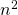in the regret bound compared to the optimistic calculations from Part 1, and thus by the end of this lecture we could still hope to prove a regret bound of order.

Gaussian core

First let us revisit the introduction of the core. Recall that we are considering a kernelsuch that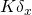is the distribution offor some random variableto be defined. To control the regret we want to have the following inequality for any convex function:which means that the distribution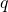of the random variableshould satisfy

(1)The core is defined so that (1) is in fact an equality. As we discussed in the previous post the core is a deep mathematical construction, but unfortunately we could not find a way to generalize the Part 1’s variance calculation when the core is non-Gaussian. In what follows we describe a slightly different construction which will allow us to satisfy (1) withbeing Gaussian. A key observation is that ifis convexly dominated by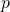, i.e. for any convexone has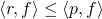, then (1) is satisfied by takingto be the core of:Thus it suffices to show that for anythat we care about one can find a Gaussian“inside” of(in the sense thatis convexly dominated by). Then instead of taking the core ofto define the kernel one can take the core of(which we will also refer to as the Gaussian core of).

Next we show that we can essentially restrict our attention to the case whereis log-concave, and then we show how to find a Gaussian inside a log-concave measure.

Approximate log-concavity

Recall that in Part 1 we replaced the variance calculation by simply showing that the loss estimatesare bounded. Thus we see by induction and using Hoeffding-Azuma that with probability at least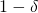one hasUsing an union bound over an-net together with Lipschitzness of(which we didn’t prove but it follows the same lines than boundedness) one has in fact that with high probability (say) there is a convex function(recall that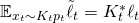is a convex function) such that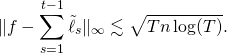In particular provided thatthe above display shows that(whose density is proportional to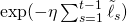) is-approximately log-concave (recall thatis said to be-approximately log-concave if there is a log-concave functionsuch that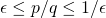). To simplify the discussion in these lectures we will in fact assume thatis exactly log-concave.

We note that the above concentration argument makes us lose a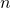factor in the regret. Indeed in Part 1 we used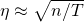to (informally) obtain the regret(or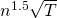with even more optimistic calculations). We see that now we are forced to take a learning rate which issmaller than this, which in turn multiplies the regret bound by a factor.

Finding a Gaussian inside a log-concave

Letbe an isotropic log-concave measure. We will show that a centered Gaussianwith covarianceis (approximately) convexly dominated by. We note in passing that by going back to the calculations at the end of Part 1 it is easy to see that this factorin the covariance will in turn force us to take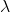to besmaller than what we hoped at the end of Part 1, leading to yet again an extrain the regret bound.

In fact we will show that any centered measuresupported on a ball of radius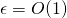is (exactly) convexly dominated by. This implies the (approximate) Gaussian convex domination we mentioned above since most of the mass ofis in such a ball (it is easy to deal with the remaining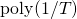mass but we won’t worry about it here).

Let us fix some convex function. We want to show that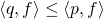. Since both measures are centered we may add any linear function towithout affecting this inequality, so we may legitimately assume thatand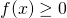for all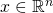. By scaling we might also assume that the maximum on the centered ball of radiusisand thus it is enough to show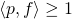. By convexityis above the linear functionwhereis the maximizer ofon the centered ball of radius. Note also that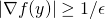and thus it is enough to show (recall that):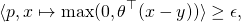where, which is implied byIt only remains to use the fact that an isotropic real log-concave random variable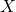verifies, and thus the above display holds true with.

This entry was posted in Optimization, Theoretical Computer Science. Bookmark the permalink.